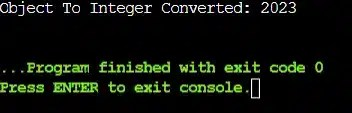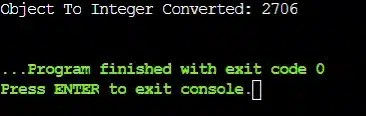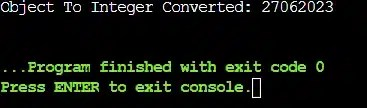# What Are The Ways To Convert Object To Int In Java? 3 Ways Explained

Do you know “How to Convert Object to Int in Java“? Ever wondered how to transform an ‘Object’ in Java and elevate it to an ‘Int’? In this article, we will talk about the possible method present to convert an object to an int value in Java.

Let us try to find out the simple method present in the Java programming language for doing such conversion of primitive data types or programming elements. Still, if you have any doubts related to converting an object to an integer in Java or issues regarding Java Code Help then you can CodingZap experts.

But before we move ahead with the demonstration of possible simple methods, we should clear our basics.

Without clearing the basic concept, it will not be a wise choice to directly jump to the core discussion. We will first look at the Object class concept before moving to convert object to int value in Java programming language.

So, let us start our discussion from the basic.

### What Is Object In Java? Read Below

So one thing should be clear in your mind that the object is the element that is present inside of the class. And with the help of the Identity, State & Behavior of types, we can distinguish those things. But before we move ahead with the conversion process, we should know its declaration process.

``````// Declaration Of Object Zap With Class One
One Zap = new One();``````

In the Java programming language, the objects are declared more oftentimes. It is a very important & frequent practice one should do while writing codes in the Object Oriented Programming concept. So, it will be better, if we look at the basic code declaration of the Object implementation.

The most common practice to declare an object is to use the ‘new’ keyword. In the above example, a new object ‘Zap’ is implemented with the help of the functions. We have used the same keyword for the creation.

We often develop an object as object obj, but here we have developed it as object zap. It is completely our choice to provide the name. Instead of Object obj, we can provide any name. It is not necessary to follow the conventional rule to provide a name as an object obj.

In the above case, the ‘Zap’ object will be developed with the help of the ‘One’ class present in the program. The concept will be cleared more with some more discussion. Now, we will move forward to know about the possible simple method for doing such a conversion.

## What Are The Methods To Convert Object To Int In Java Programming Language?

We should all be aware that, due to many reasons oftentimes we need to convert object to int value in the program. In the Java programming language, there are three methods possible for doing such a conversation. And it is good news that each & every method follows a simple way & easy to implement.

The lists of methods that are helpful in such situations are the following:

• Using Wrapper Class
• Using intValue() Method
• Using parseInt() Method

We will all know about all of these one by one briefly along with the respective code in the Java programming language. It will help to get a good grip on the topic.

### Convert an Object To Int In Java Using Wrapper ClassOverview of the process to write the code:

• At the very beginning of the program, we will create an object inside of the default main class. That means we will start our program implementation from the public static void main. The object implementation will be the first step.
• After the declaration of the object (as we have seen while the object definition part), we will move to the primitive int value-assigning stage. That means we will work on some integers.
• Here one new integer variable will be declared & some random primitive type int values will be provided. That value will be then transferred to the object value. Else, we can directly provide the int value to the object.
• Now, another function has been created by us to make the process easier. But the same thing can be implemented in the default static main also. We will call that method where the created object will be shared as the argument value.
• Inside of that, we will use the wrapper class. That means, the term ‘Integer’ will be provided in the brackets just before the object name. Hence, the object value has now again been converted to the int value. Then we will return the integer value to the default main.
• Inside the static main, we will print the data that has come as integer data. So, the integer representation of the object value will be completed. The proper example you find is the following program.

General Syntax: (Integer)object-name

##### Code to convert obj to int using wrapper class:

``````public class Main { public static int con(Object Zap){
int w = (Integer)Zap; // Implementing Wrapper Class
return w;}
public static void main(String args[]) {
Object Zap=new Object(); // Creation Of Object
Zap=2023; // Assigning Object Value
int i=con(Zap);
System.out.println("Object To Integer Converted: " + i);}}``````
###### Steps of Code:
1. First, the declaration of the public static void main() should be done.
2. Inside of that, the creation of the object (here the term ‘Zap’) will be done.
3. After that, the int value will be assigned to the object value. Here, the value is 2023.
4. Another function will be called along with the object name as the argument in the function.
5. The object will convert to an int value by using the syntax. And the data will return.

Let us try to find out the output of the above code. It will help to understand the method to convert object to int in the Java programming language using the wrapper class.

##### Output:Here, the output of the program clearly shows that the object value has now been converted to an integer. And the code is error-free because it is terminated with exit code 0.

### Convert Object To Int In Java Using intValue() Method

Step by Step overview of the code

• The intValue() is an inbuilt method present in the Java programming language. The method is used to convert any primitive data type to the integer or number value in the program. We will use the concept in this case.
• Here, the initial flow of the program will be the same as we have done in the above example. The declaration steps of the public static void main() method will be the same as we have done earlier. But still, we are giving an overview of it due to the easy understandability of the process.
• Here, the program again starts from the development of the static main. Inside the static main, we should again create an object using the ‘new’ keyword. After the declaration of the object, we will provide some int value to the object.
• In Java, static keyword plays an important role as when it is used before the function name, it becomes a static function. To know more about how it is used in C++ programming language, you can check out our article here
• Hence, the integer value will become the object value. The same object value should convert to the int value. For that purpose, another method has been declared. The object value will be shared as the argument to the method.
• Inside of that, we will take the object value. And one special syntax statement will be executed by the program. The syntax is quite difficult to explain, we will use one minus sign there. But the syntax ends with the intValue() function. Thus, it will convert the object value to the integer format.
• The data will return to the static main method. And the value will be printed there. The proper example you find is the following program.

General Syntax: ((Number)Object-Name).intValue()

##### Code to convert obj to int using intValue() method:

``````public class Main { public static int con(Object Zap){
int x = ((Number)Zap).intValue(); // Using The intValue() Function
return x;}
public static void main(String args[]) {
Object Zap=new Object(); // Creation Of Object
Zap=2706; int a=con(Zap);
System.out.println("Object To Integer Converted: " + a);}}``````
###### Step by step of Code:

1. The public static void main will be the starting point. So, it should be developed first.
2. Create the object inside the main function. We have used the ‘new’ keyword for that purpose.
3. Assign some random int value to the object & share the object value with the method as an argument.
4. The intValue() statement will be developed in the program & the data will return for printing.

Let us try to find out the output of the above code. It will help to understand the conversion method of the object to an integer in the Java programming language using the intValue() function.

##### Output:As the program is giving output, it is clear that the program executed perfectly. And the output helps to know that the object value is successfully converted to the int value.

### What Is The Way To Convert an Object To Int In Java Using The parseInt() Method?

Understand the Overview of Code

• parseInt() is also another inbuilt method present in the Java programming language. Here, anything that is provided as the argument in this method will be converted to the int value. But for doing an object-to-integer conversion process, we will use one tricky line.
• Here, the program will again start with the public static void main statement. And the declaration of the method will be the same as we have done earlier for those two cases. So, we will not focus now on that part. Rather move to another implementation.
• While using the parseInt() function, the object value will be first converted to the string object. As the object-to-string conversion is very easy, it will not take any separate statement. Along with the syntax, we can do the operation. To gain more knowledge about strings in Java, you can know about comparing strings in Java.
• The data will return to the main method for printing purposes. The proper example you find is the following program.

General Syntax: Integer.parseInt(Object-Name.toString());

##### Code to convert obj to int using ParseInt() method:

``````public class Main { public static int con(Object Zap){
int x = Integer.parseInt(Zap.toString()); // Using The parseInt() Function
return x;}
public static void main(String args[]) {
Object Zap=new Object();
int v =27062023; // Assigning Integer Value
Zap=v; // Assigning Object Value
int a=con(Zap); System.out.println("Object To Integer Converted: " + a);}}``````
###### Steps of Code:

1. First, the declaration of the public static void main() should be done.
2. Inside Of that, an object will be created. And one integer value will be declared.
3. After that, the integer value will be assigned to the object value.
4. Another method will be called along with the object name as the argument in the function.
5. The conversion will be done using the above-mentioned syntax & the data will return & be printed.

Let us try to find out the output of the above code. It will help to understand the conversion method of an object to an integer in the Java programming language using the parseInt() function.

##### Output:Here, the output shows that it is concluded by sharing the exit code zero with the compiler. And the method is successful as mentioned by this error-free output.

## Conclusion:

As we saw, we should know the process to convert Object to Int in Java programming language.

We have discussed three possible ways in this topic, those are used for this conversion process. Among those three, whatever is best suited to you that can be used inside of the Java programming language without any problem.

It is advisable to clear the basic concept in the Java programming language along with the integer class concept.  In Java, arrays also play an important role, and learning about methods of printing an array in Java will help you gain more knowledge in this field as it helps us to declare the value of an integer.

If the basic concept is cleared, then such a high-level topic will become a cakewalk for any student. So, you should focus on your basic knowledge of Java programming language.